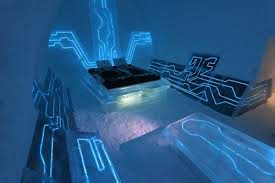Published on Sep 16, 2019

### Abstract

The Objective : This project is a series of three experiments that test the effects of distance on the intensity of radioactive sources and the effects of mass absorption of beta and gamma radiation.

### Methods/Materials

1. Spectrum Techniques ST-360 Counter with GM Tube (Power supply, stand, clamp, USB cable, source holder, and 20 shields of varying mass thickness.)

2. Sony Notebook Computer

3. Radioactive Sources: Beta Sr-90 & Gamma Co-60

The procedure for the Inverse Square Law experiment is to place the detector on the clamp of the ruler stand. Then, place the radioactive source on the surface of a table. Record 10 readings at 60 second intervals for each source from 2cm to 24cm at 1cm intervals. The procedures for the Beta and Gamma experiments are to place the source in the source holder inside the shelf stand 3cm away from the detector. Next, place a shield 2cm away from the detector. Record 10 readings at 60 second intervals for each source and each shield.

### Results

The results for The Inverse Square Law experiment and the Beta and Gamma experiments are: Graphs A and C describe intensity as inversely proportional to the square of the distance and plot as a hyperbola. Graphs B and D describe intensity as proportional to the reciprocal of the square of the distance and plot as a straight line. Graphs E and G graph as a decrease in intensity as the mass thickness of the absorbers is increased.

Graphs F and H graph intensity logarithmically verses the increasing shield numbers.

The beta graph begins as a straight line but intensity decreases rapidly by aluminum shield M. Graph H plots as a straight line with a downward slope as the mass thickness of the shield numbers increase until the thickest lead absorber T is used.

Gamma radiation is more penetrating than beta radiation, and lead is a better absorber of aluminum when one compares their mass thickness. The half thickness was calculated to be 6553 mg/cm^2 for gamma.

### Conclusions/Discussion

Experimentation partially supports my objectives, goals, and results of the Inverse Square Law and the Beta and Gamma Absorption experiments.

The project states the intensity of radiation decreases proportionally as the inverse square of the distance, and the effectiveness of radioactive shielding improves with materials of increasing density and linear thickness.